# 用AI给黑白照片上色，复现记忆中的旧时光

【导读】我们知道，深度学习几乎已经应用在每一个领域，但如果我们能够构建一个基于深度学习的模型，让它能够给老照片着色，重现我们童年的旧回忆，这该多么令人激动啊！那么我们要怎么做呢？本文的作者将为大家介绍一个教程，通过深度学习方法为黑白老照片自动上色，带我们重新忆起那段老时光！

1. AI技术的易用性、性价比、安全性都有待考量和实验
2. AI难落地，云虽然是AI的载体，但现在的云厂商所能提供的AI能力非常有限
3. 开发者自身难转型，难适应的问题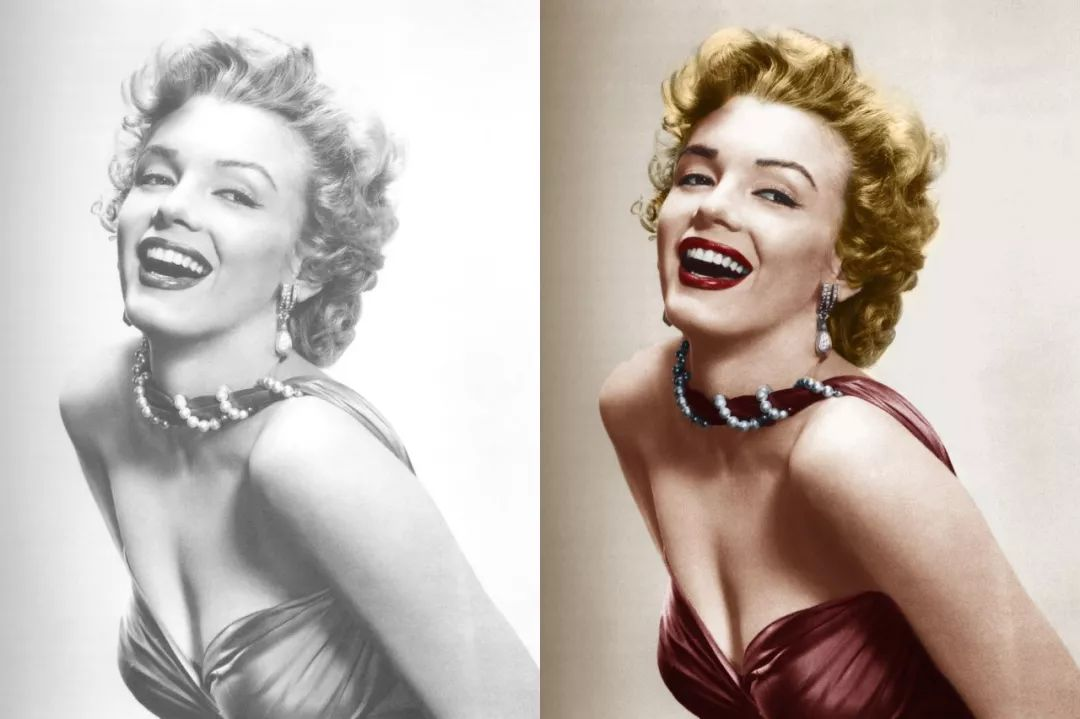### 介绍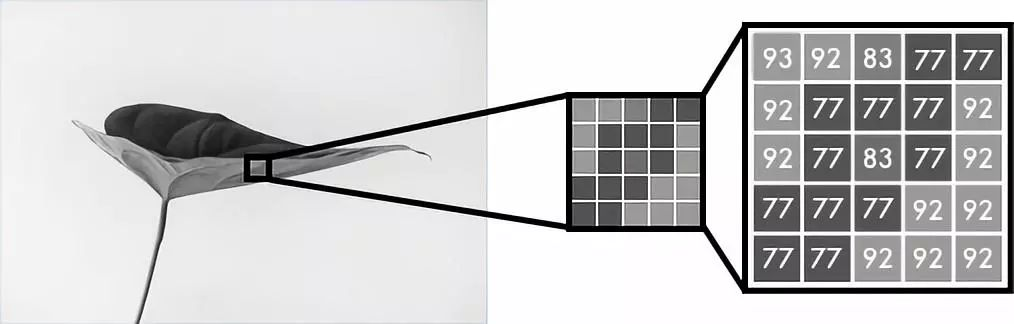﻿﻿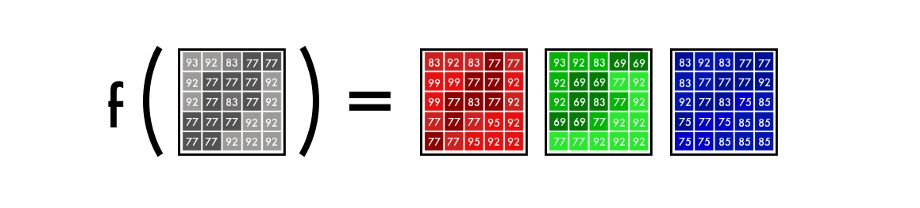f()是神经网络，[B&W]是我们的输入，[R]、[G]、[B]是我们的输出

Deep Learning made easy with Deep Learning Studio — An Introduction

Deep Learning made easy with Deep Learning Studio — Complete Guide

A video walkthrough of Deep Cognition

python代码和数据集可以从 GitHub 中下载

### 环境设置

Deep Learning Studio 最好的地方之一就是，只需单击 Deep Learning Studio Cloud，就可以轻松地完成安装，然后随时随地使用它们。

1.安装 Python 环境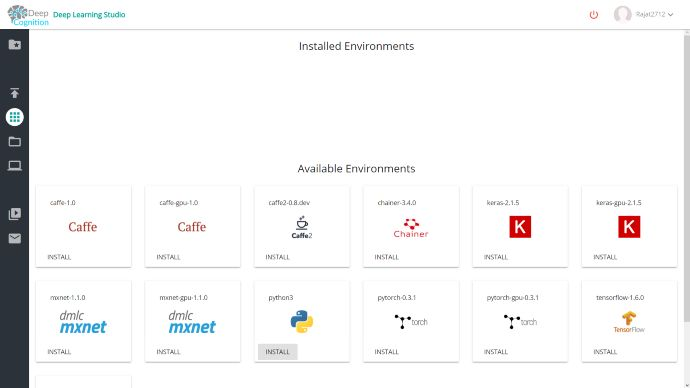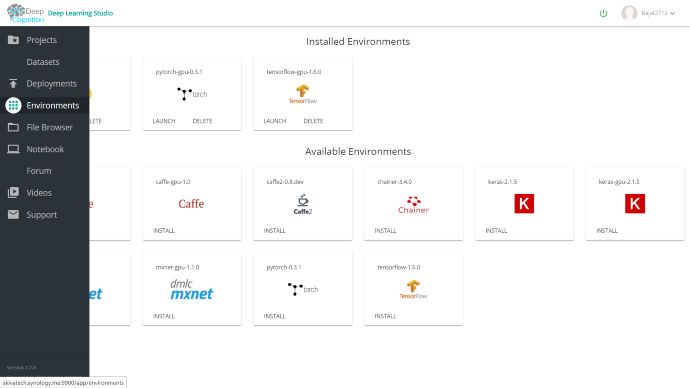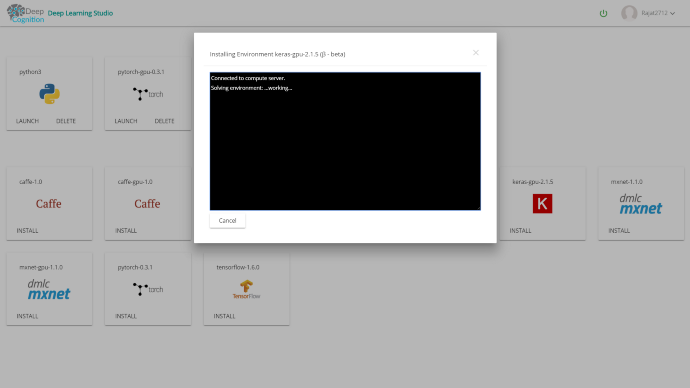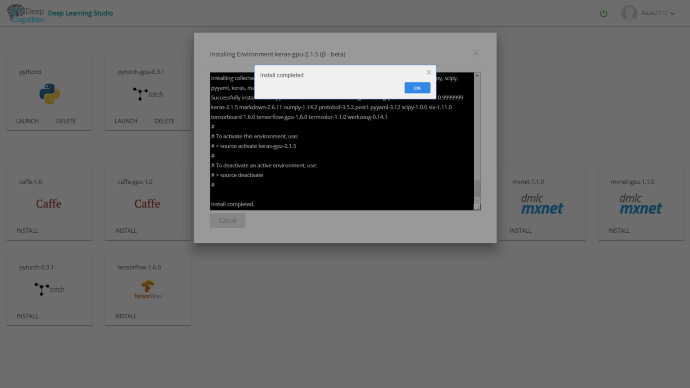• Python3

• Tensorflow-gpu-1.6.0

• Keras-gpu-2.1.5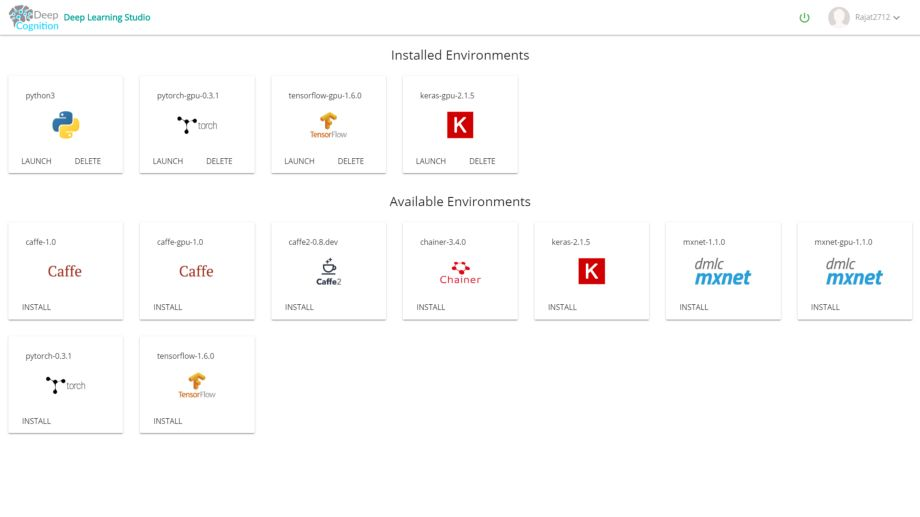2.安装python包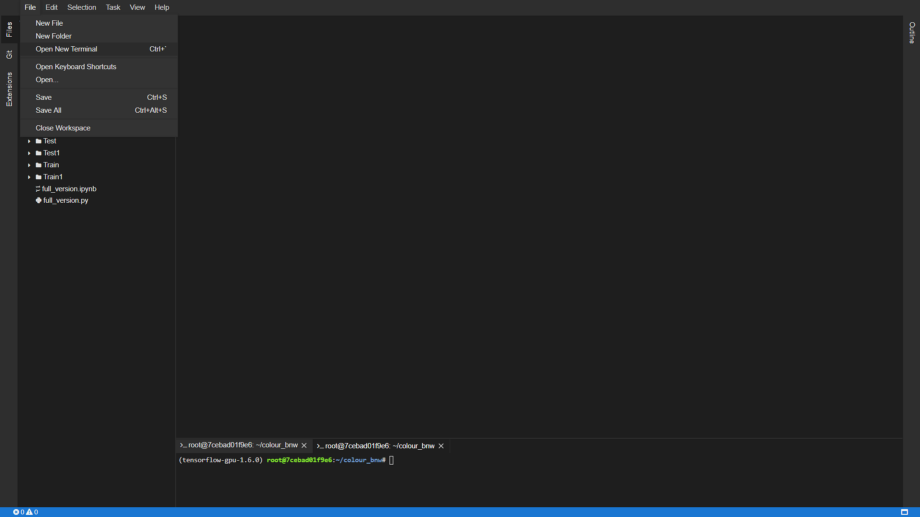1pip install scikit-image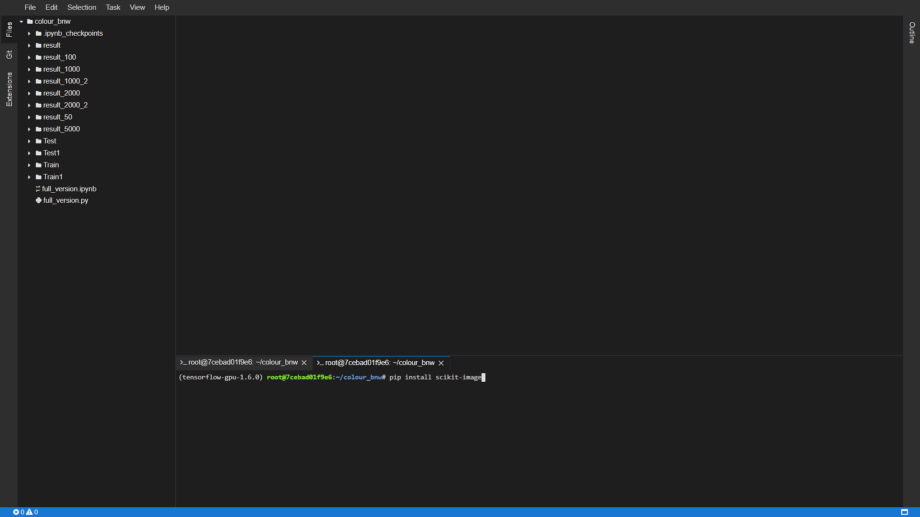### 接下来开始编码

 1import keras
2from keras.applications.inception_resnet_v2 import InceptionResNetV2
3from keras.preprocessing import image
4from keras.engine import Layer
5from keras.applications.inception_resnet_v2 import preprocess_input
6from keras.layers import Conv2D, UpSampling2D, InputLayer, Conv2DTranspose, Input, Reshape, merge, concatenate
7from keras.layers import Activation, Dense, Dropout, Flatten
8from keras.layers.normalization import BatchNormalization
9from keras.callbacks import TensorBoard
10from keras.models import Sequential, Model
11from keras.layers.core import RepeatVector, Permute
12from keras.preprocessing.image import ImageDataGenerator, array_to_img, img_to_array, load_img
13from skimage.color import rgb2lab, lab2rgb, rgb2gray, gray2rgb
14from skimage.transform import resize
15from skimage.io import imsave
16import numpy as np
17import os
18import random
19import tensorflow as tf



1# Get images
2X = []
3for filename in os.listdir('Train/'):
5X = np.array(X, dtype=float)
6Xtrain = 1.0/255*X
8inception = InceptionResNetV2(weights='imagenet', include_top=True)
9inception.graph = tf.get_default_graph()


Inception ResNet v2 是一个在120万张图像上训练的神经网络，也是现今最强大的分类器之一。与编码器并行，输入图像也通过 Inception ResNet v2 来运行。提取分类层并将其与编码器的输出合并。

 1embed_input = Input(shape=(1000,))
2#Encoder
3encoder_input = Input(shape=(256, 256, 1,))
4encoder_output = Conv2D(64, (3,3), activation='relu', padding='same', strides=2)(encoder_input)
5encoder_output = Conv2D(128, (3,3), activation='relu', padding='same')(encoder_output)
6encoder_output = Conv2D(128, (3,3), activation='relu', padding='same', strides=2)(encoder_output)
7encoder_output = Conv2D(256, (3,3), activation='relu', padding='same')(encoder_output)
8encoder_output = Conv2D(256, (3,3), activation='relu', padding='same', strides=2)(encoder_output)
9encoder_output = Conv2D(512, (3,3), activation='relu', padding='same')(encoder_output)
10encoder_output = Conv2D(512, (3,3), activation='relu', padding='same')(encoder_output)
11encoder_output = Conv2D(256, (3,3), activation='relu', padding='same')(encoder_output)
12#Fusion
13fusion_output = RepeatVector(32 * 32)(embed_input)
14fusion_output = Reshape(([32, 32, 1000]))(fusion_output)
15fusion_output = concatenate([encoder_output, fusion_output], axis=3)
16fusion_output = Conv2D(256, (1, 1), activation='relu', padding='same')(fusion_output)
17#Decoder
18decoder_output = Conv2D(128, (3,3), activation='relu', padding='same')(fusion_output)
19decoder_output = UpSampling2D((2, 2))(decoder_output)
20decoder_output = Conv2D(64, (3,3), activation='relu', padding='same')(decoder_output)
21decoder_output = UpSampling2D((2, 2))(decoder_output)
22decoder_output = Conv2D(32, (3,3), activation='relu', padding='same')(decoder_output)
23decoder_output = Conv2D(16, (3,3), activation='relu', padding='same')(decoder_output)
24decoder_output = Conv2D(2, (3, 3), activation='tanh', padding='same')(decoder_output)
25decoder_output = UpSampling2D((2, 2))(decoder_output)
26model = Model(inputs=[encoder_input, embed_input], outputs=decoder_output)


﻿现在，我们必须调整图像的大小来适应 Inception 模型。然后根据模型对像素和颜色值使用预处理器进行格式化。在最后一步中，我们通过 Inception 网络运行它并提取模型的最后一层。

 1def create_inception_embedding(grayscaled_rgb):
2    grayscaled_rgb_resized = []
3    for i in grayscaled_rgb:
4        i = resize(i, (299, 299, 3), mode='constant')
5        grayscaled_rgb_resized.append(i)
6    grayscaled_rgb_resized = np.array(grayscaled_rgb_resized)
7    grayscaled_rgb_resized = preprocess_input(grayscaled_rgb_resized)
8    with inception.graph.as_default():
9        embed = inception.predict(grayscaled_rgb_resized)
10    return embed


﻿用 ImageDataGenertor 可以调整图像生成器的设置。如此一来得到不会重复的图像，从而提高了学习率。shear_rangetilts 使图像向左或向右倾斜，其他设置为缩放、旋转和水平翻转。

1# Image transformer
2datagen = ImageDataGenerator(
3        shear_range=0.2,
4        zoom_range=0.2,
5        rotation_range=20,
6        horizontal_flip=True)
7#Generate training data
8batch_size = 10


﻿我们使用 Xtrain 文件夹中的图像，根据上面的设置生成图像。然后，为 X_batch 提取黑色层和白色层，并为两个颜色层提取两种颜色。

1def image_a_b_gen(batch_size):
2    for batch in datagen.flow(Xtrain, batch_size=batch_size):
3        grayscaled_rgb = gray2rgb(rgb2gray(batch))
4        embed = create_inception_embedding(grayscaled_rgb)
5        lab_batch = rgb2lab(batch)
6        X_batch = lab_batch[:,:,:,0]
7        X_batch = X_batch.reshape(X_batch.shape+(1,))
8        Y_batch = lab_batch[:,:,:,1:] / 128
9        yield ([X_batch, create_inception_embedding(grayscaled_rgb)], Y_batch)


GPU 越强，得到的图像就越多。通过现在的设置，你可以使用50~100张图像。steps_per_epoch 是通过将训练图像的数量除以 batch 大小来计算的。

1#Train model
2model.compile(optimizer='rmsprop', loss='mse')
3model.fit_generator(image_a_b_gen(batch_size), epochs=50, steps_per_epoch=1)


﻿1.0/255 表示我们使用的是 24 位 RGB 颜色空间，这意味着我们为每个颜色通道使用 0 ~ 255 之间的数字。这将会产生 1670 万种颜色的组合。

1color_me = []
2for filename in os.listdir('Test/'):
4color_me = np.array(color_me, dtype=float)
5gray_me = gray2rgb(rgb2gray(1.0/255*color_me))
6color_me_embed = create_inception_embedding(gray_me)
7color_me = rgb2lab(1.0/255*color_me)[:,:,:,0]
8color_me = color_me+.reshape(color_me.shape+(1,))


﻿神经网络进行训练后，做出最终的预测，并将其转化为图像。

1# Test model
2output = model.predict([color_me, color_me_embed])
3output = output * 128
4# Output colorizations
5for i in range(len(output)):
6    cur = np.zeros((256, 256, 3))
7    cur[:,:,0] = color_me[i][:,:,0]
8    cur[:,:,1:] = output[i]
9    imsave("result/img_"+str(i)+".png", lab2rgb(cur))


### ﻿结果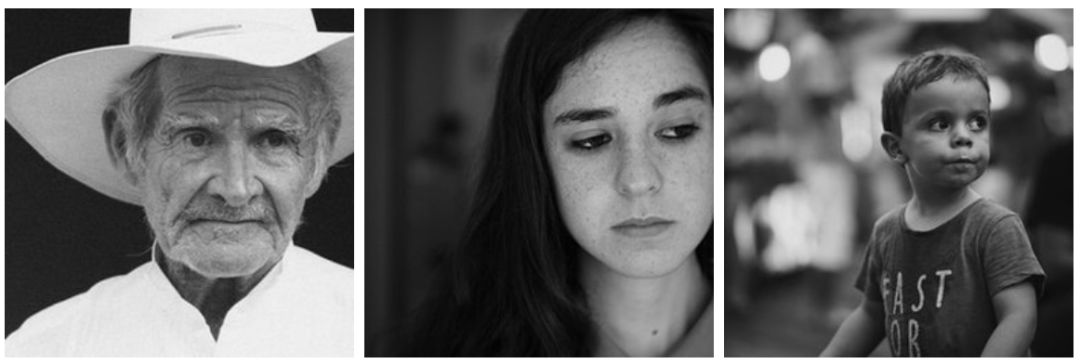﻿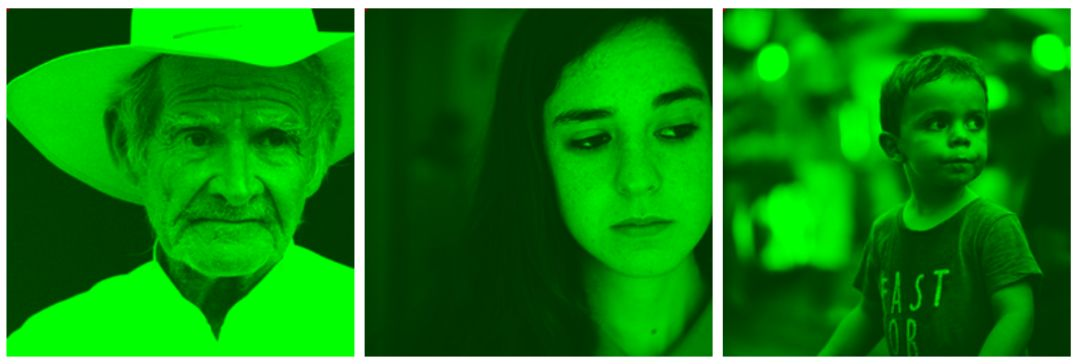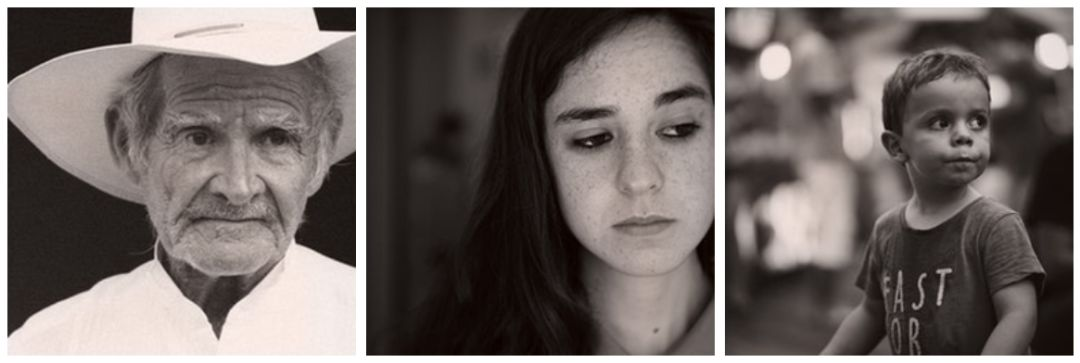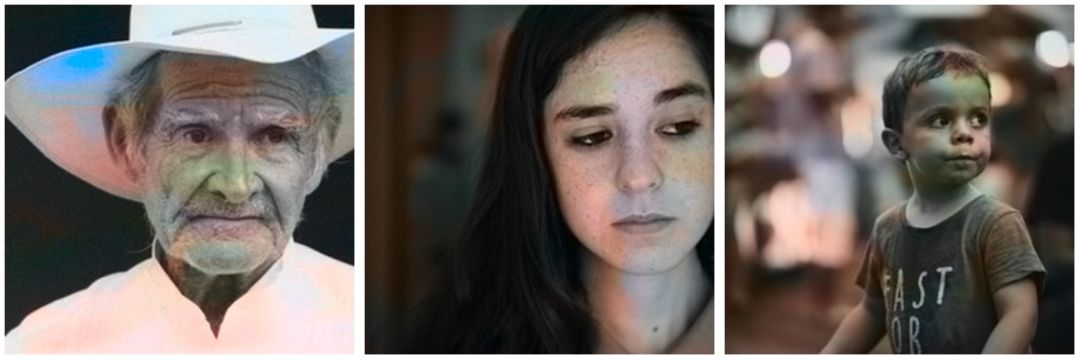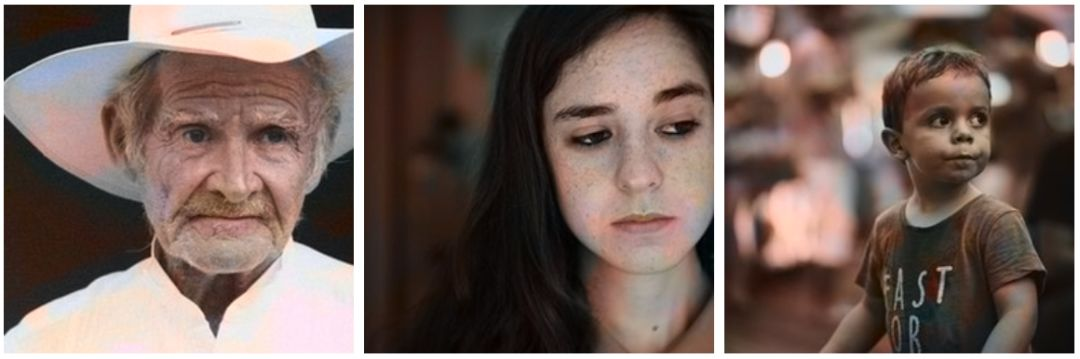—【完】—

2018 AI开发者大会

2018 AI开发者大会是一场由中美人工智能技术高手联袂打造的AI技术与产业的年度盛会！是一场以技术落地为导向的干货会议！大会设置了10场技术专题论坛，力邀15+硅谷实力讲师团和80+AI领军企业技术核心人物，多位一线经验大咖带你将AI从云端落地。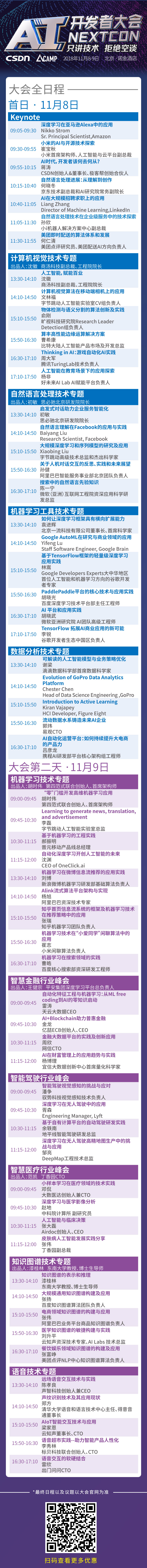04-15148
07-066490

01-273188
12-291701
09-20327
08-271552
07-211万+
09-181万+
12-292689
05-133290
11-2571
10-242932
02-191157
07-051万+
06-078502
10-281万+
11-303338
11-08233
03-251214
09-09355

### “相关推荐”对你有帮助么？

•非常没帮助
•没帮助
•一般
•有帮助
•非常有帮助点击重新获取扫码支付余额充值